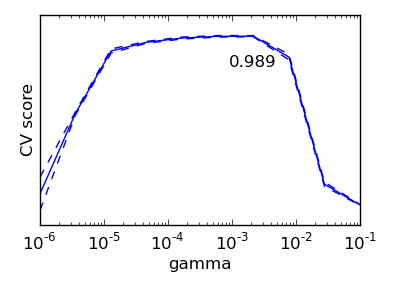# 3. Model selection: choosing estimators and their parameters¶

## 3.1. Score, and cross-validated scores¶

As we have seen, every estimator exposes a score method that can judge the quality of the fit (or the prediction) on new data. Bigger is better.

```>>> from scikits.learn import datasets, svm
>>> X_digits = digits.data
>>> y_digits = digits.target
>>> svc = svm.SVC()
>>> svc.fit(X_digits[:-100], y_digits[:-100]).score(X_digits[-100:], y_digits[-100:])
0.97999999999999998
```

To get a better measure of prediction accuracy (which we can use as a proxy for goodness of fit of the model), we can successively split the data in folds that we use for training and testing:

```>>> import numpy as np
>>> X_folds = np.array_split(X_digits, 10)
>>> y_folds = np.array_split(y_digits, 10)
>>> scores = list()
>>> for k in range(10):
...     # We use 'list' to copy, in order to 'pop' later on
...     X_train = list(X_folds)
...     X_test  = X_train.pop(k)
...     X_train = np.concatenate(X_train)
...     y_train = list(y_folds)
...     y_test  = y_train.pop(k)
...     y_train = np.concatenate(y_train)
...     scores.append(svc.fit(X_train, y_train).score(X_test, y_test))
>>> print scores
[0.9555555555555556, 1.0, 0.93333333333333335, 0.99444444444444446, 0.98333333333333328, 0.98888888888888893, 0.99444444444444446, 0.994413407821229, 0.97206703910614523, 0.96089385474860334]
```

This is called a K-Fold cross-validation.

## 3.2. Cross-validation generators¶

The code above to split data in train and test sets is tedious to write. The scikits.learn exposes cross-validation generators to generate list of indices for this purpose:

```>>> from scikits.learn import cross_val
>>> k_fold = cross_val.KFold(n=6, k=3)
Train: [False False  True  True  True  True] | test: [ True  True False False False False]
Train: [ True  True False False  True  True] | test: [False False  True  True False False]
Train: [ True  True  True  True False False] | test: [False False False False  True  True]
```

The cross-validation can then be implemented easily:

```>>> kfold = cross_val.KFold(len(X_digits), k=3)
>>> [svc.fit(X_digits[train], y_digits[train]).score(X_digits[test], y_digits[test])
...          for train, test in kfold]
[0.95530726256983245, 1.0, 0.93296089385474856, 0.98324022346368711, 0.98882681564245811, 0.98882681564245811, 0.994413407821229, 0.994413407821229, 0.97206703910614523, 0.95161290322580649]
```

To compute the score method of an estimator, the scikits.learn exposes a helper function:

```>>> cross_val.cross_val_score(svc, X_digits, y_digits, cv=kfold, n_jobs=-1)
array([ 0.95530726,  1.        ,  0.93296089,  0.98324022,  0.98882682,
0.98882682,  0.99441341,  0.99441341,  0.97206704,  0.9516129 ])
```

n_jobs=-1 means that the computation will be dispatched on all the CPUs of the computer.

Cross-validation generators
 KFold(n, k) StratifiedKFold(y, k) LeaveOneOut(n) LeaveOneLabelOut(labels) Split it K folds, train on K-1, test on left-out Make sure that all classes are even accross the folds Leave one observation out Takes a label array to group observationsExercise

On the digits dataset, plot the cross-validation score of a SVC estimator with an RBF kernel as a function of gamma (use a logarithmic grid of points, from 1e-6 to 1e-1).

## 3.3. Grid-search and cross-validated estimators¶

### 3.3.2. Cross-validated estimators¶

Cross-validation to set a parameter can be done more efficiently on an algorithm-by-algorithm basis. This is why, for certain estimators, the scikits.learn exposes “CV” estimators, that set their parameter automatically by cross-validation:

```>>> from scikits.learn import linear_model, datasets
>>> lasso = linear_model.LassoCV()
>>> X_diabetes = diabetes.data
>>> y_diabetes = diabetes.target
>>> lasso.fit(X_diabetes, y_diabetes)
>>> # The estimator chose automatically its lambda:
>>> lasso.alpha
0.0075421928471338063
```

These estimators are called similarly to their counterparts, with ‘CV’ appended to their name.

Exercise

On the diabetes dataset, find the optimal regularization parameter alpha.

Bonus: How much can you trust the selection of alpha?